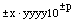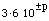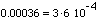Date: Wed, 7 Oct 1998 09:18:21 -0700 (PDT) Help! How do you express the following numbers in scientific(exponential)notation:0.00036 and 2998000000. I understand how to solve them if it was an equation, but this is confusing me. Please help me answer my questions. Thank you. Hi, A number written in scientic notation means the number is expressed aswhere the x's, y's are digits, x is larger than 0 and p is a positive integer. The number of y's in the expression depends on the number of significant digits in the number. For your first number 0.00036, the scientific notation isfor some p. Since to get 0.00036 from 3.6 you need to move the decimat 4 places to the left,Now try 2998000000. Cheers, Harley

Go to Math Central